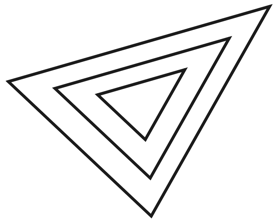Geometry Demystified, 2nd edition Stan Gibilisco Explanations for Quiz Answers in Chapter 2 1. Choices A, B, and C constitute statements that always hold true for triangles. However, choice D can sometimes turn out false. You can find these situations described, in detail, on page 28 of the text. If two triangles have corresponding interior angles with equal measures as you go around them both in the same direction, then those triangles exhibit direct similarity, but not necessarily direct congruence. One triangle might be larger than the other one (and therefore have longer sides), even though the two triangles' corresponding angles have identical measures. The answer is D. As a matter of fact, if you choose any set of three interior-angle measures, you can construct infinitely many triangles whose corresponding interior angles have those measures. In the drawing below, for example, all three triangles are directly similar; you can doubtless imagine infinitely many more triangles directly similar to, but larger or smaller than, these three.2. A casual glance at the triangle in Figure 2-15 reveals an obtuse angle at the top, and acute angles at the lower left and lower right. Because this triangle has one obtuse angle, it's an obtuse triangle by definition. If you don't want to rely on your eyes alone, you can use a protractor (an instrument for measuring angles, available at most good stationery stores) to physically measure the angle at the top. You'll come out with a value larger than 90º but smaller than 180º, as it should be for an obtuse angle. The correct choice is B. 3. If you want to find the area of a triangle, you can multiply its base length by half its height. In Figure 2-15, you can call line segment RP the base, so the shortest distance between point Q and line segment RP equals the height. The correct choice is D. If you look back at Figure 2-14 on page 40 of the text, you'll see that Figure 2-15 shows the same sort of situation (except that the points have different names). 4. An equilateral triangle always has three identical interior angles; they all measure 60º. Therefore, if you take any two equilateral triangles and go around them in opposite directions, you'll find that the corresponding angles have equal measures. Choice C makes a true statement. Choices A and B, however, both make statements that do not always hold true. If either A or B were valid statements, then all equilateral triangles would have equal sizes as well as identical shapes. But, of course, equilateral triangles come in all sizes. The answer is C. 5. We can use the side-angle-side (SAS), side-side-side (SSS), and angle-side-angle (ASA) principles to establish direct congruence between two triangles. However, the angle-angle-angle (AAA) principle can only establish direct similarity, not direct congruence. The correct choice is C. 6. In Figure 2-16, we see a 90º angle (a right angle) between the sides measuring s and h. Therefore, the triangle whose sides have lengths s, h, and v constititutes a right triangle by definition. The answer is B. 7. The interior area of a triangle equals the base length times half the height. In the situation of Figure 2-16, the base length equals (t + s), and the height equals h. We can therefore calculate the area A using the formula A = (t + s) h / 2 This formula doesn't appear as any of our choices! However, we can multiply out the numerator in the right-hand side of the above equation, "morphing" it into A = (th + sh) / 2 and then, taking advantage of the commutative laws of addition and multiplication, "morph" it some more to obtain A = (hs + ht) / 2 That's choice D. 8. The perimeter of a triangle equals the sum of the lengths of its sides. In the situation of Figure 2-16, the sides of the large triangle, defined by the vertices Q, R, and P, measure (t + s), u, and v. Therefore, we can calculate the perimeter B as B = (t + s) + u + v= s + t + u + v The correct choice is B. 9. We've seen that the triangle in Figure 2-16 whose sides measure s, h, and v is a right triangle. In this case, v is the hypotenuse, so the Pythagorean theorem tells us that v 2 = s 2 + h 2 The correct choice is A. 10. When we examine Figure 2-16, we can see that the triangle whose sides measure t, h, and u is a right triangle; the side of length u is the hypotenuse. We know it's a right triangle because the angle between the sides of lengths t and h must be 90º (it adds to the 90º angle between the sides of lengths s and h to get a straight angle of 180º). Therefore u 2 = h 2 + t 2 according to the Pythagorean theorem. When we subtract t 2 from both sides of the foregoing equation and then transpose the left-hand and right-hand sides, we get h 2 = u 2 - t 2 The answer is C.